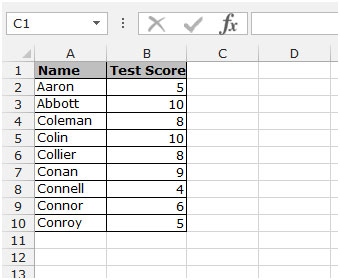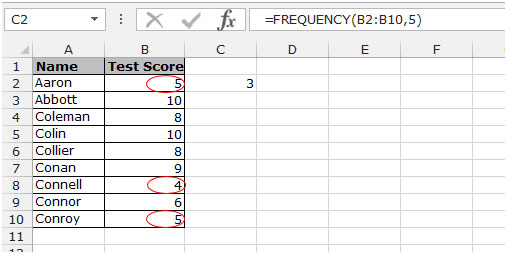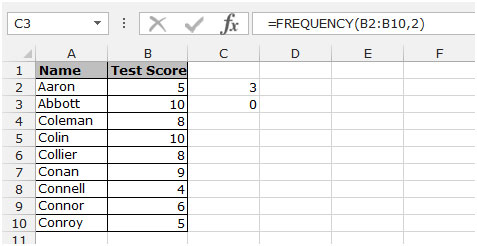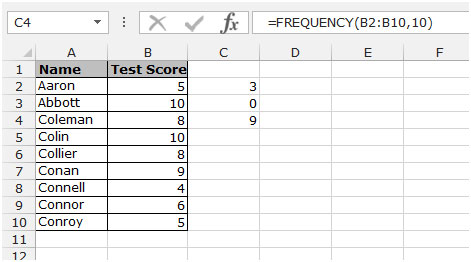# FREQUENCY : This function calculates the amount of data in a given interval and then returns a vertical array of numbers with one more element than the binary array.

In this section we will learn how and where we can use the Frequency function in Microsoft Excel2010 and 2013 .

## How to use the FREQUENCY function:

= FREQUENCY (data_array, bins_array)

Let’s take an example to better understand how and where we can use the Frequency function in Microsoft Excel2010 and 2013 .

## To create the Frequency formula, enter the array into Excel

Example 1: We have data in the range A1: B10 . Column A contains the name and column B contains the test score.For example, in this data we want to check the frequency of 5, to calculate the frequency you must follow the steps below:

• Select cell C2 and write the formula.
• = FREQUENCY (B2: B10.5)

Enter and then press enter .

• This function returns the value 3, ie there are 3 numbers whose value is from zero to 5 (picture below).Let’s calculate the frequency of number 2 this time, to check the frequency of number 2, follow the steps below:

• Select cell C3 and write the formula.

= FREQUENCY (B2: B10.2)

• Enter and then press enter .
• This function returns the number 0, meaning that there are 0 numbers up to 2.This time let’s calculate the frequency of 10, select cell C4 and write the formula.

= FREQUENCY (B2: B10,10)

• Enter and then press enter .
• This function returns 9, that is, there are 9 numbers until 10.Well, this is a way to check the data frequency using the Frequency function in Excel 2010 and 2013.